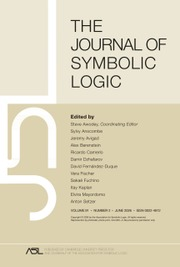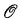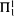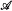HomeThe Journal of Symbolic Logic

# Π11 relations and paths throughPublished online by Cambridge University Press:  12 March 2014

Corresponding

## Extract

When bounds on complexity of some aspect of a structure are preserved under isomorphism, we refer to them as intrinsic. Here, building on work of Soskov , , we give syntactical conditions necessary and sufficient for a relation to be intrinsicallyon a structure. We consider some examples of computable structuresand intrinsicallyrelations R. We also consider a general family of examples of intrinsicallyrelations arising in computable structures of maximum Scott rank.

For three of the examples, the maximal well-ordered initial segment in a Harrison ordering, the superatomic part of a Harrison Boolean algebra, and the height-possessing part of a Harrison p-group, we show that the Turing degrees of images of the relation in computable copies of the structure are the same as the Turing degrees ofpaths through Kleene's. With this as motivation, we investigate the possible degrees of these paths. We show that there is apath in which ∅′ is not computable. In fact, there is one in which no noncomputable hyperarithmetical set is computable. There are paths that are Turing incomparable, or Turing incomparable over a given hyperarithmetical set. There is a pair of paths whose degrees form a minimal pair. However, there is no path of minimal degree.

Type
Research Article
Information
The Journal of Symbolic Logic , June 2004 , pp. 585 - 611

## Access options

Get access to the full version of this content by using one of the access options below.

## References

Ash, C. J. and Knight, J. F., Possible degrees in recursive copies, Annals of Pure and Applied Logic, vol. 75 (1995), pp. 215221.CrossRefGoogle Scholar
Ash, C. J. and Knight, J. F., Possible degrees in recursive copies II, Annals of Pure and Applied Logic, vol. 87 (1997), pp. 151165.CrossRefGoogle Scholar
Ash, C. J. and Knight, J. F., Computable structures and the hyperarithmetical hierarchy, Elsevier, 2000.Google Scholar
Ash, C. J., Knight, J. F., Manasse, M., and Slaman, T., Generic copies of countable structures, Annals of Pure and Applied Logic, vol. 42 (1989), pp. 195205.CrossRefGoogle Scholar
Ash, C. J. and Nerode, A., Intrinsically recursive relations, Aspects of effective algebra (Crossley, J. N., editor), Steel's Creek, Australia, Upside Down A Book Co., 1981, pp. 2641.Google Scholar
Barker, E., Intrinsicallyrelations, Annals of Pure and Applied Logic, vol. 39 (1988), pp. 105130.CrossRefGoogle Scholar
Barwise, J., Infinitary logic and admissible sets, this Journal, vol. 34 (1969), pp. 226252.Google Scholar
Chisholm, J., Effective model theory vs. recursive model theory, this Journal, vol. 55 (1990), pp. 11681191.Google Scholar
Downey, R., Jockusch, C. G. Jr., and Stob, M., Array nonrecursive degrees and genericity, Computability, enumerability, unsolvability, London Mathematical Society Lecture Notes Series, vol. 224, Cambridge University Press, 1996, pp. 93104.CrossRefGoogle Scholar
Friedman, H., One hundred and two problems in mathematical logic, this Journal, vol. 40 (1975), pp. 113129.Google Scholar
Friedman, H., Recursiveness inpaths through, Proceedings of the American Mathematical Society, vol. 54 (1976), pp. 311315.Google Scholar
Goncharov, S. S., The quantity of nonautoequivalent constructivizations, Algebra and Logic, vol. 16 (1977), pp. 169185, English translation.CrossRefGoogle Scholar
Goncharov, S. S., Harizanov, V. S., Knight, J. F., McCoy, C., Miller, R. G., and Solomon, R., Enumerations in computable structure theory, submited.Google Scholar
Goncharov, S. S. and Knight, J. F., Computable structure and non-structure theorems, Algebra and Logic, vol. 41 (2002), pp. 351373, English translation.CrossRefGoogle Scholar
Harizanov, V. S., Some effects of Ash-Nerode and other decidability conditions on degree spectra, Annals of Pure and Applied Logic, vol. 55 (1991), pp. 5165.CrossRefGoogle Scholar
Harrington, L., McLaughlin's conjecture, handwritten notes, 1976.Google Scholar
Harrison, J., Recursive pseudo-well-orderings, Transactions of the American Mathematical Society, vol. 131 (1968), pp. 526543.CrossRefGoogle Scholar
Hirschfeldt, D. R., Khoussainov, B., Shore, R. A., and Slinko, A. M., Degree spectra and computable dimensions in algebraic structures, Annals of Pure and Applied Logic, vol. 115 (2002), pp. 71113.CrossRefGoogle Scholar
Jockusch, C. G. Jr., Recursiveness of initial segments of Kleene's, Fundamenta Mathematical vol. 87 (1975), pp. 161167.CrossRefGoogle Scholar
Jockusch, C. G. Jr., and McLaughlin, T. G., Countable retracing functions andpredicates, Pacific Journal of Mathematics, vol. 30 (1969), pp. 6793.CrossRefGoogle Scholar
Kaplansky, I., Infinite abelian groups, University of Michigan Press, 1954.Google Scholar
Khousainov, B. and Shore, R. A., Computable isomorphisms, degree spectra of relations and Scott families, Annals of Pure and Applied Logic, vol. 93 (1998), pp. 153193.CrossRefGoogle Scholar
Kreisel, G., Set theoretic problems suggested by the notion of potential totality, Infinitistic methods, Proceedings of the Symposium on Foundations of Mathematics, Warsaw, 1959, Pergamon, 1961, pp. 103140.Google Scholar
Lachlan, A. H., Solution to a problem of Spector, Canadian Journal of Mathematics, vol. 23 (1971), pp. 247256.CrossRefGoogle Scholar
Lerman, M., Degrees of unsolvability, Springer-Verlag, Berlin, 1983.CrossRefGoogle Scholar
Manasse, M. S., Techniques and counterexamples in almost categorical recursive model theory, Ph.D. thesis, University of Wisconsin-Madison, 1982.Google Scholar
Parikh, R., A note on paths through, Proceedings of the American Mathematical Society, vol. 39 (1973), pp. 178180.Google Scholar
Ressayre, J. P., Models with compactness properties relative to an admissible language, Annals of Mathematical Logic, vol. 11 (1977), pp. 3155.CrossRefGoogle Scholar
Rogers, H. Jr., Theory of recursive functions and effective computability, McGraw-Hill, New York, 1967.Google Scholar
Sacks, G. E., On the number of countable models, Southeast Asian Conference on Logic (Singapore, 1981) (Chong, C. T. and Wicks, M. J., editors), North-Holland, 1983, pp. 185195.CrossRefGoogle Scholar
Sacks, G. E., Higher recursion theory, Springer-Verlag, 1990.CrossRefGoogle Scholar
Scott, D., Logic with denumerably long formulas and finite strings of quantifiers, The theory of models (Addison, J. W., Henkin, L., and Tarski, A., editors), Proc. 1963 Internat. Sympos. Berkeley, North-Holland, 1965, pp. 329341.Google Scholar
Soskov, I. N., Intrinsically hyperarithmetical sets, Mathematical Logic Quarterly, vol. 42 (1996), pp. 469480.CrossRefGoogle Scholar
Soskov, I. N., Intrinsicallyrelations, Mathematical Logic Quarterly, vol. 42 (1996), pp. 109126.CrossRefGoogle Scholar
Spector, C., Recursive well-orderings, this Journal, vol, 20 (1955), pp. 151163.Google Scholar
Steel, J. R., Forcing with tagged trees, Annals of Mathematical Logic, vol. 15 (1978), pp. 5574.CrossRefGoogle Scholar

### Full text views

Full text views reflects PDF downloads, PDFs sent to Google Drive, Dropbox and Kindle and HTML full text views.

Total number of HTML views: 0
Total number of PDF views: 15 *
View data table for this chart

* Views captured on Cambridge Core between September 2016 - 19th January 2021. This data will be updated every 24 hours.

Hostname: page-component-76cb886bbf-2sjx4 Total loading time: 0.583 Render date: 2021-01-19T14:48:29.882Z Query parameters: { "hasAccess": "0", "openAccess": "0", "isLogged": "0", "lang": "en" } Feature Flags: { "shouldUseShareProductTool": true, "shouldUseHypothesis": true, "isUnsiloEnabled": true, "metricsAbstractViews": false, "figures": false, "newCiteModal": false }

# Send article to Kindle

Note you can select to send to either the @free.kindle.com or @kindle.com variations. ‘@free.kindle.com’ emails are free but can only be sent to your device when it is connected to wi-fi. ‘@kindle.com’ emails can be delivered even when you are not connected to wi-fi, but note that service fees apply.

Find out more about the Kindle Personal Document Service.

Π11 relations and paths throughAvailable formats × # Send article to Dropbox To send this article to your Dropbox account, please select one or more formats and confirm that you agree to abide by our usage policies. If this is the first time you use this feature, you will be asked to authorise Cambridge Core to connect with your <service> account. Find out more about sending content to Dropbox. Π11 relations and paths throughAvailable formats
×

# Send article to Google Drive

To send this article to your Google Drive account, please select one or more formats and confirm that you agree to abide by our usage policies. If this is the first time you use this feature, you will be asked to authorise Cambridge Core to connect with your <service> account. Find out more about sending content to Google Drive.

Π11 relations and paths throughAvailable formats
×
×